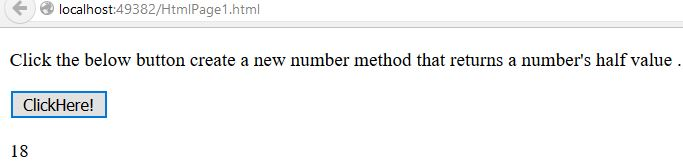# Number prototype Property in JavaScript

##### How to create a new number method that returns a number's half value in JavaScript?

By using `Number.prototype` property we can create a new number method that returns a number's calculated value.

```<p>Click the below button create a new number method that returns a number's half value .</p>
<input type="button" value="ClickHere!" onclick="HalfNumber()" />
<p id="myId"></p>

<script>
Number.prototype.myMethod = function () {
return this.valueOf() / 2;
}

function HalfNumber() {
var A = 36;
document.getElementById("myId").innerHTML = A.myMethod();
}
</script>
```

In the above code snippet we have given `Id` as "`myId`"to the second `<p>` element in the HTML code. There is a function HalfNumber() in the`<script>`block which is connected to the Onclick of the HTML button, we need to create a new number method, for that we are using `Number.prototype` and for returning the number's half value we are using `return this.valueOf() / 2`The `document.getElementById(`"myId"`) ` returns the element that has Id "id=myID" in the HTML page. Onclick of the button "ClickHere!" fires the function HalfNumber() in the `<script>` block, at the same time the `number.prototype` creates a new number method and `return this.valueOf() / 2` returns the number's half value as output.

OUTPUTNOTE: If you want to create a new number method that returns a number's quarter value we can use `return this.valueOf() / 4`.

```<script>
Number.prototype.myMethod = function () {
return this.valueOf() / 4;
}

function HalfNumber() {
var A = 36;
document.getElementById("myId").innerHTML = A.myMethod();
}
</script>
```

Dividing & finding the 4th part

In the above code snippet we have divided the number into 4 parts.

Views: 3415 | Post Order: 86